# Solving Rational Equations and Inequalities Algebra 2 8

• Slides: 32Solving Rational Equations and Inequalities Algebra 2 8 -5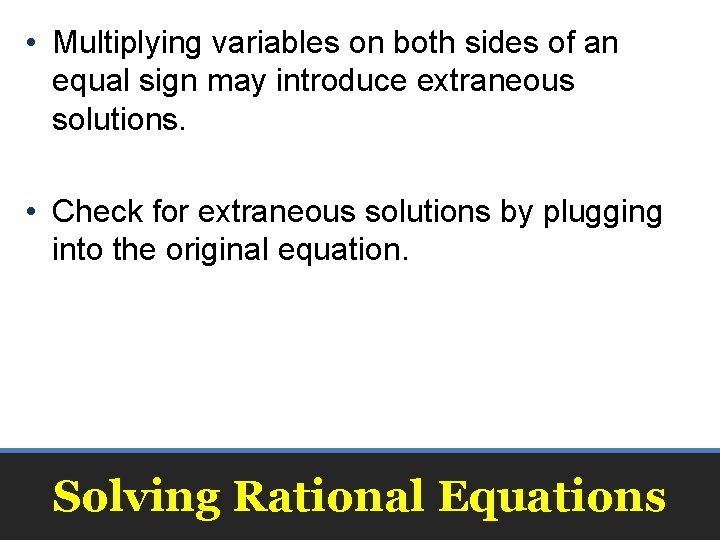• Multiplying variables on both sides of an equal sign may introduce extraneous solutions. • Check for extraneous solutions by plugging into the original equation. Solving Rational EquationsSolving Rational Equations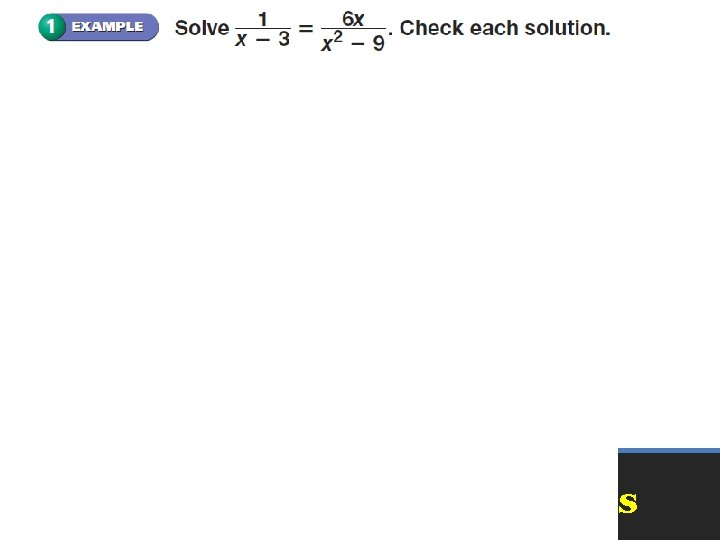Solving Rational EquationsSolving Rational Equations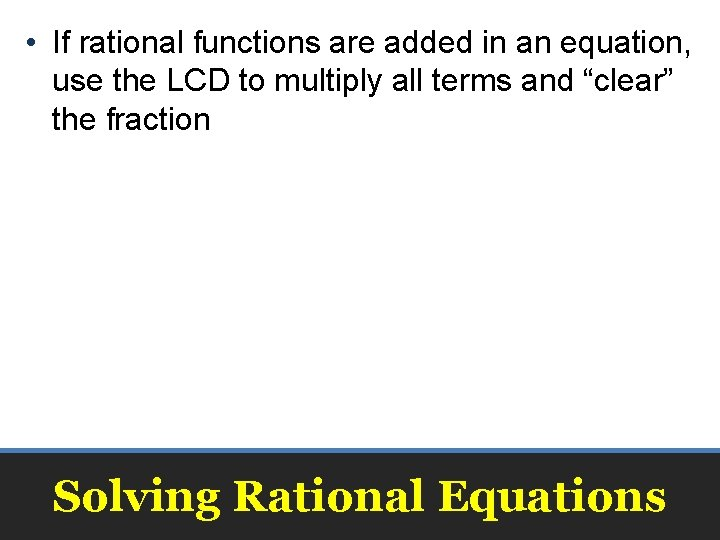• If rational functions are added in an equation, use the LCD to multiply all terms and “clear” the fraction Solving Rational EquationsSolving Rational Equations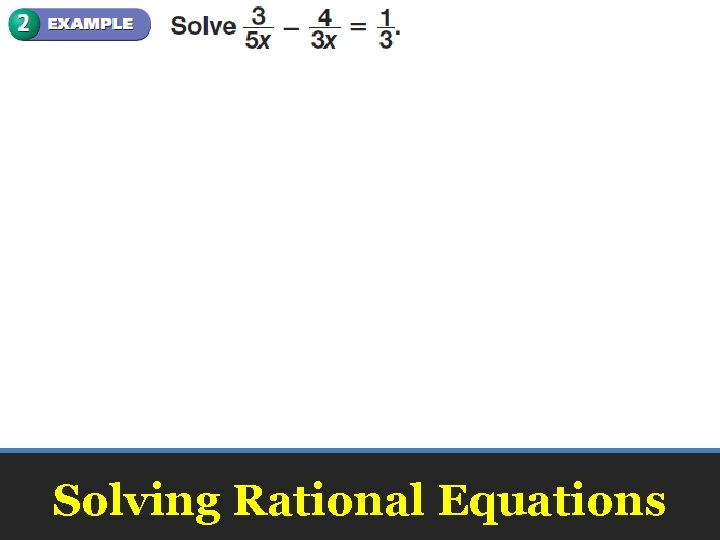Solving Rational Equations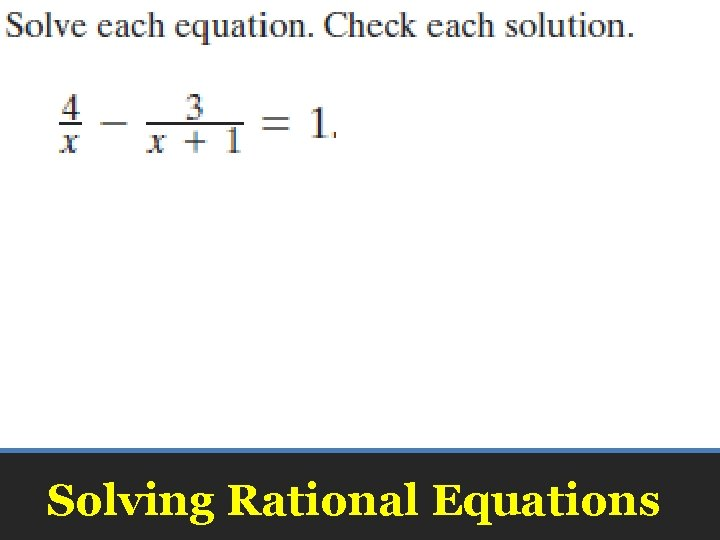Solving Rational Equations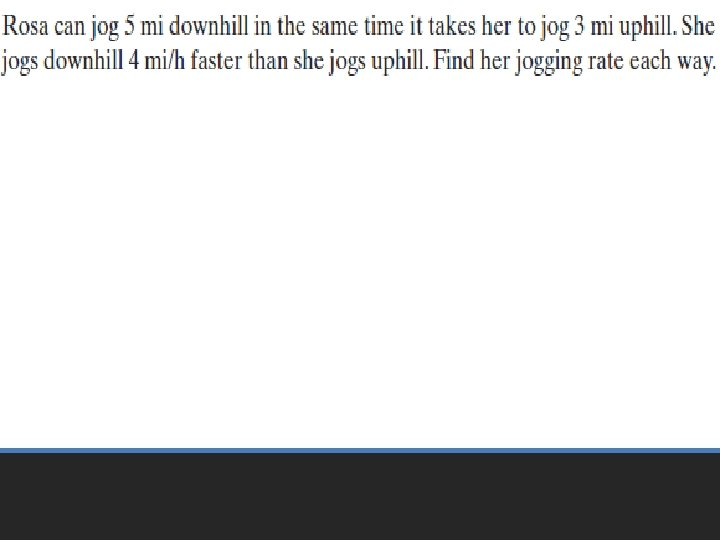Mowing Lawns Tim and Ashley mow lawns together. Tim working alone could complete the job in 4. 5 hours, and Ashley could complete it alone in 3. 7 hours. How long does it take to complete the job when they work together? In 1 hour, Tim could complete In 1 hour, Ashley could complete of the job. Solving Rational Equations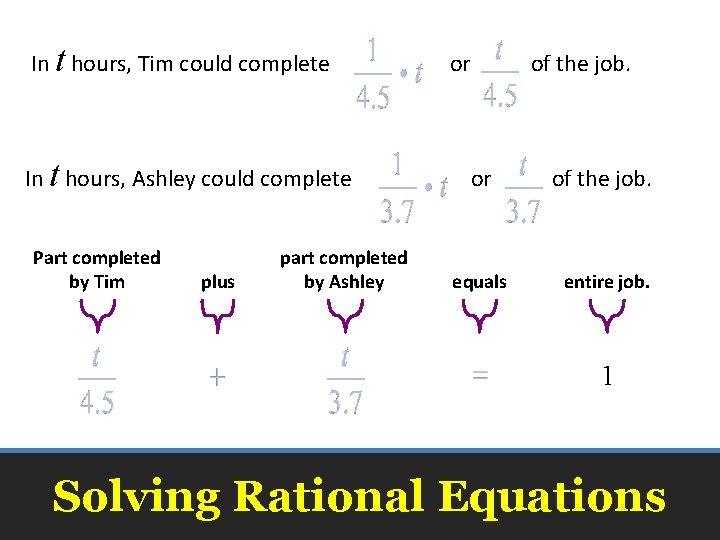In t hours, Tim could complete In t hours, Ashley could complete Part completed by Tim plus part completed by Ashley or of the job. equals entire job. 1 Solving Rational Equations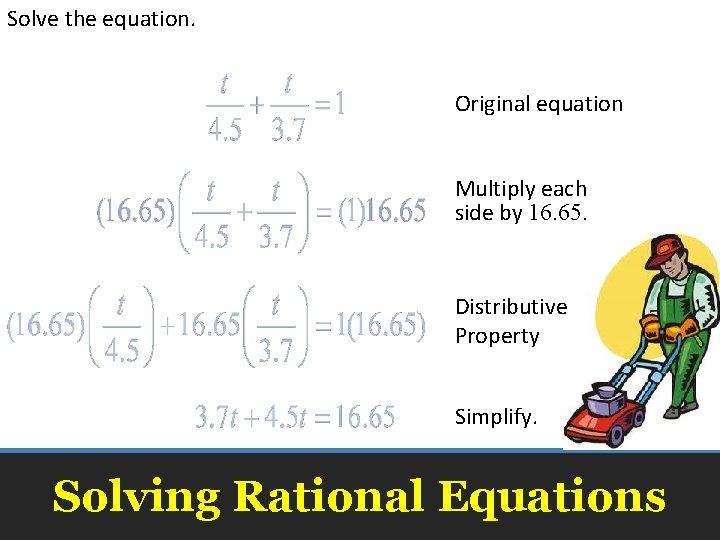Solve the equation. Original equation Multiply each side by 16. 65. Distributive Property Simplify. Solving Rational Equations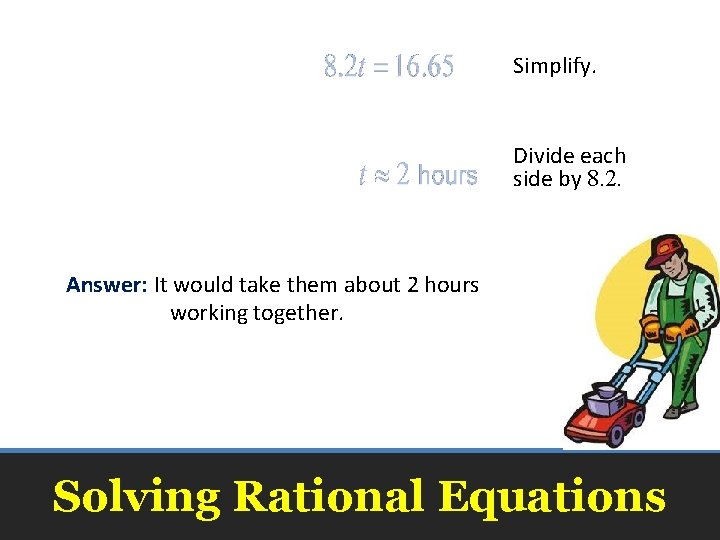Simplify. Divide each side by 8. 2. Answer: It would take them about 2 hours working together. Solving Rational EquationsCleaning Libby and Nate clean together. Nate working alone could complete the job in 3 hours, and Libby could complete it alone in 5 hours. How long does it take to complete the job when they work together? about 2 hours Solving Rational Equations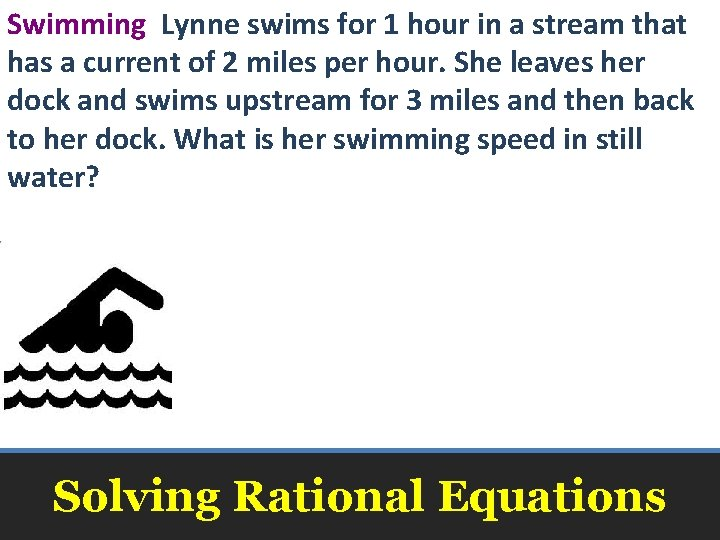Swimming Lynne swims for 1 hour in a stream that has a current of 2 miles per hour. She leaves her dock and swims upstream for 3 miles and then back to her dock. What is her swimming speed in still water? about 6. 6 mph Solving Rational EquationsSolve Step 1 Values that make the denominator equal to 0 are excluded from the denominator. For this inequality the excluded value is 0. Step 2 Solve the related equation. Related equation Solving Rational Equations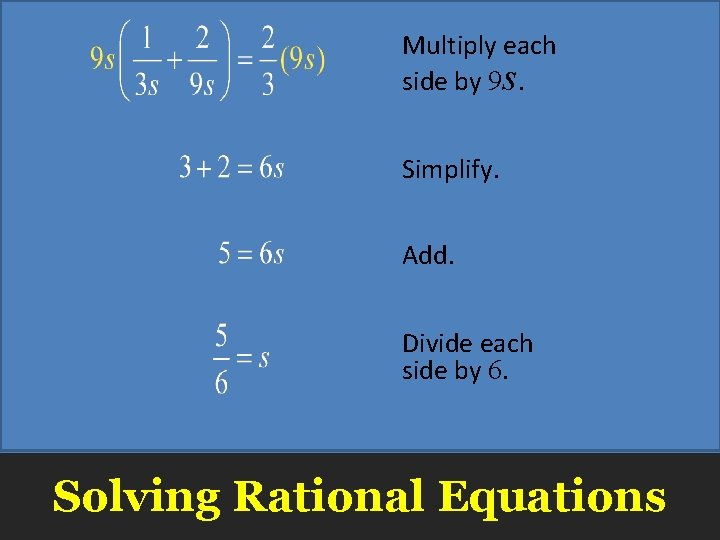Multiply each side by 9 s. Simplify. Add. Divide each side by 6. Solving Rational EquationsStep 3 Draw vertical lines at the excluded value and at the solution to separate the number line into regions. Now test a sample value in each region to determine if the values in the region satisfy the inequality. Solving Rational Equations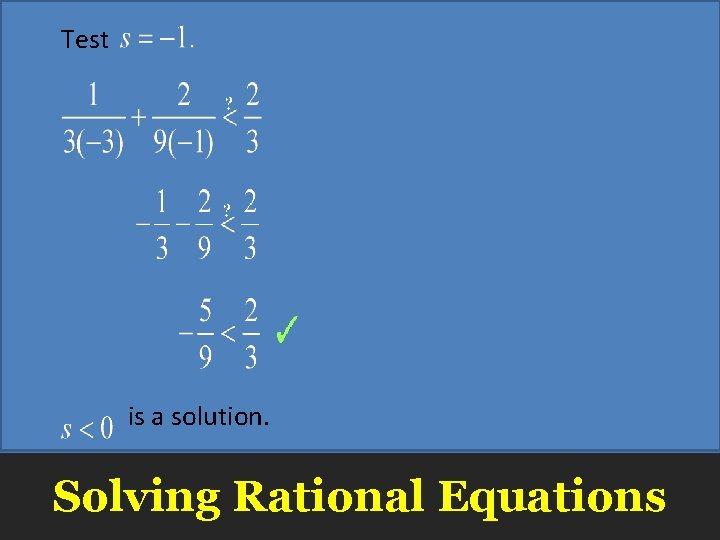Test is a solution. Solving Rational Equations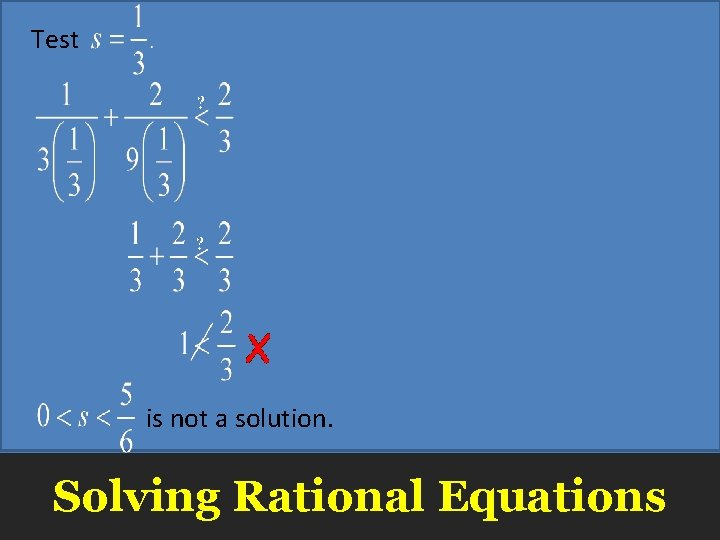Test is not a solution. Solving Rational EquationsTest is a solution. Solving Rational Equations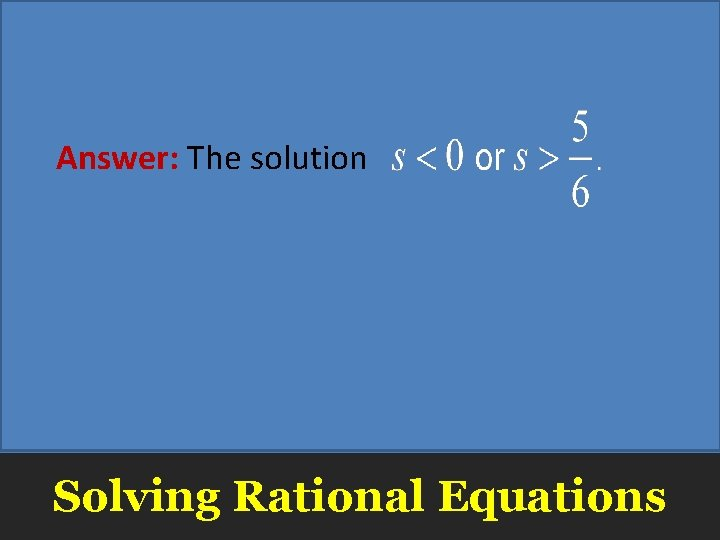Answer: The solution Solving Rational EquationsSolve Answer: Solving Rational Equations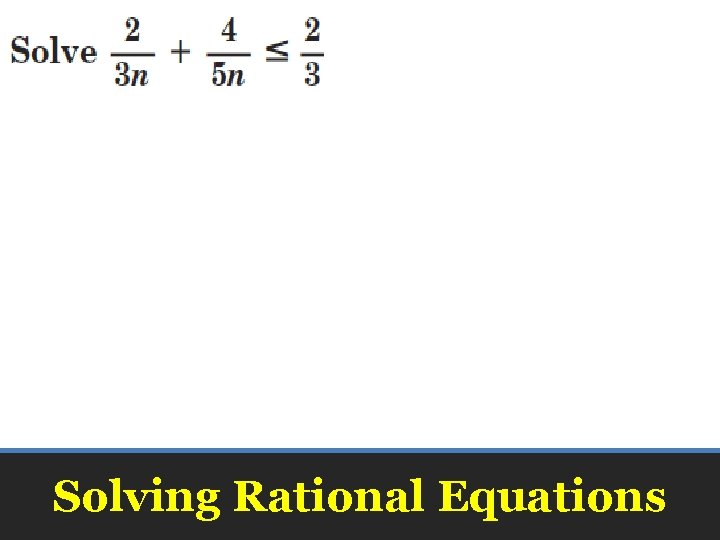Solving Rational Equations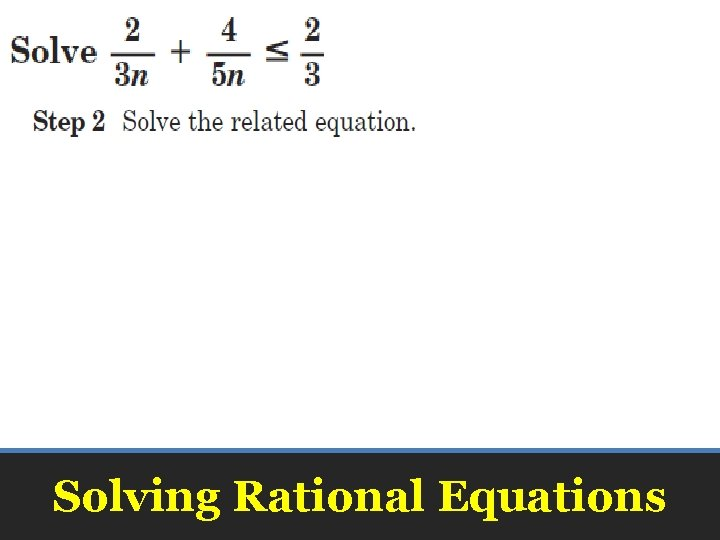Solving Rational Equations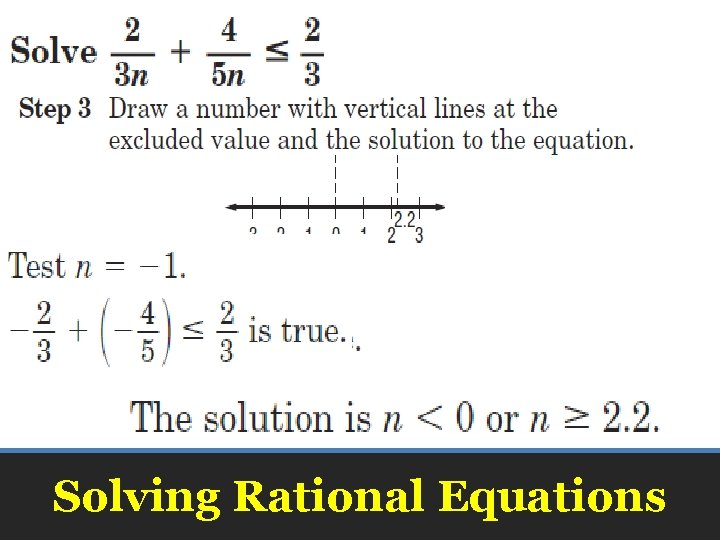Solving Rational Equations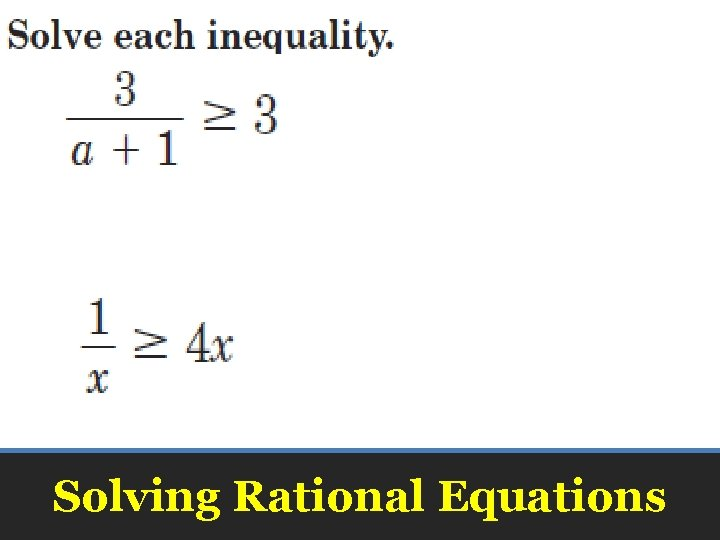Solving Rational EquationsSolving Rational Equations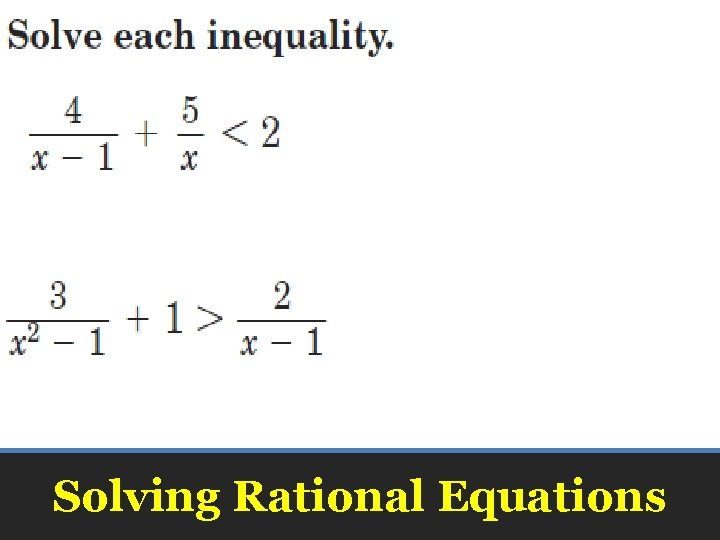Solving Rational Equations• Pages 605 – 606 • 20 – 26 even, 38 – 42 even, 48, 50 Homework